Next: General Solution of the Up: Klein-Gordon Equation Previous: Lorentz Invariance

# Solutions of the Klein-Gordon Equation

We look for plane-wave solutions with both positive and negative energy. They will form a complete set. We try (with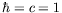)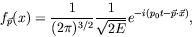(4.16)

where we have chosen an arbitrary normalization for now. These forms are eigenfunctions of the operates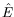and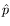with eigenvalues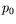and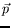, respectively. The choice of sign in the exponential is arbitrary since the Klein-Gordon equation is second order in the derivatives. Our convention is in accordance with the nonrelativistic theory.

Operating with the Klein-Gordon operator on these wave functions gives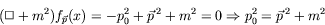(4.17)

or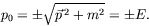(4.18)

For, we have positive-energy solutions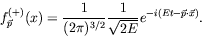(4.19)

For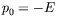, it is standard to reverse the arbitrary sign convention and write the negative-energy solutions as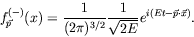(4.20)

This enables us to write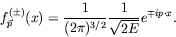(4.21)

The positive-energy solutions thus have momentum eigenvalues, while the negative-energy solutions have momentum eigenvalues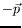. The positive- and negative-energy solutions will not mix amongst each other. Once a state is an eigenstate of one type of energy it will not become an eigenstate of the other.

Notice that many different normalizations of the wave function are in use. Some common amplitudes for plane waves are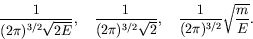(4.22)

The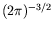factor is common in the continuum language and it is often replaced in the box normalization convention by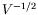, where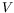is the volume of a box in which the physical interaction is confined. For the remainder of this chapter we use the first normalization given in equation 4.22.Next: General Solution of the Up: Klein-Gordon Equation Previous: Lorentz Invariance
Douglas M. Gingrich (gingrich@ ualberta.ca)
2004-03-18